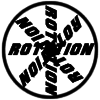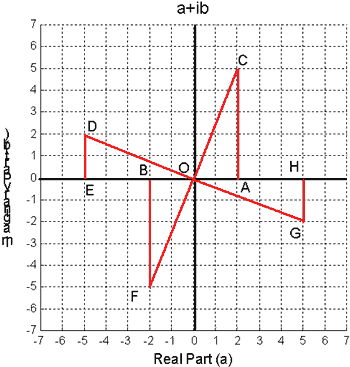#### You may also like### 8 Methods for Three by One

This problem in geometry has been solved in no less than EIGHT ways by a pair of students. How would you solve it? How many of their solutions can you follow? How are they the same or different? Which do you like best?### Rots and Refs

Follow hints using a little coordinate geometry, plane geometry and trig to see how matrices are used to work on transformations of the plane.### Reflect Again

Follow hints to investigate the matrix which gives a reflection of the plane in the line y=tanx. Show that the combination of two reflections in intersecting lines is a rotation.

# The Matrix

##### Age 16 to 18Challenge Level
Congratulations Andrei Lazanu, age 14, of School No. 205, Bucharest, Romania for this excellent solution.

I looked at the images of points (1, 0) and (0, 1) respectively, under the transformation by the matrix $$\left( \begin{array}{cc} a & b \\ c & c \end{array} \right)$$ $$\left( \begin{array}{cc} a & b \\ c & d \end{array} \right) \left( \begin{array}{c} 1 \\ 0 \end{array} \right) = \left( \begin{array}{c} a \\ c \end{array} \right)$$

This means that under this transformation, the point (1, 0) maps to the point $(a,c)$. $$\left( \begin{array}{cc} a & b \\ c & d \end{array} \right) \left( \begin{array}{c} 0 \\ 1 \end{array} \right) = \left( \begin{array}{c} b \\ d \end{array} \right)$$ Under this transformation, the point (0,1) maps to the point $(b,d)$.

Now, I look at the effect on the plane of the four transformations:

1. $b = c = 0,\ a = d = -1$ $$\left( \begin{array}{cc} -1 & 0 \\ 0 & -1 \end{array} \right) \left( \begin{array}{c} x \\ y \end{array} \right) = \left( \begin{array}{c} -x \\ -y \end{array} \right) .$$ This means each point (x, y) transforms into its symmetrical image with respect to the origin, i.e. into the point (-x, -y). This is a rotation of 180 degrees about the origin.

2. $b = c = 0,\ a = - 1,\ d = 1$ $$\left( \begin{array}{cc} -1 & 0 \\ 0 & 1 \end{array} \right) \left( \begin{array}{c} x \\ y \end{array} \right) = \left( \begin{array}{c} -x \\ y \end{array} \right) .$$ Here, it is a reflection in the y-axis.

3. $b = c = 0,\ a = d = 1$ $$\left( \begin{array}{cc} 1 & 0 \\ 0 & 1 \end{array} \right) \left( \begin{array}{c} x \\ y \end{array} \right) = \left( \begin{array}{c} x \\ y \end{array} \right) .$$ In this situation each point transforms into itself.

4. $b = c = 0,\ a = 1,\ d = -1$ $$\left( \begin{array}{cc} 1 & 0 \\ 0 & -1 \end{array} \right) \left( \begin{array}{c} x \\ y \end{array} \right) = \left( \begin{array}{c} x \\ -y \end{array} \right) .$$ This transformation leaves the abscissa unchanged and modifies the sign of the ordinate, being a reflection in the x-axis.

Now, I look at the next set of transformations.

5. $a = d = 0,\ b = c = 1$ $$\left( \begin{array}{cc} 0 & 1 \\ 1 & 0 \end{array} \right) \left( \begin{array}{c} x \\ y \end{array} \right) = \left( \begin{array}{c} y \\ x \end{array} \right) .$$ In this transformation, the abscissa and the ordinate are interchanged, the transformation being a reflection in respect to the line $y=x$, the angle bisector of the first quadrant.

6. $a = d = 0,\ b = 1,\ c = -1$ $$\left( \begin{array}{cc} 0 & 1 \\ -1 & 0 \end{array} \right) \left( \begin{array}{c} x \\ y \end{array} \right) = \left( \begin{array}{c} y \\ -x \end{array} \right) .$$ Looking to the solution to 'Complex Rotations' from the NRICH +15, July 2003, I see that this comes to the transformation of point C into point G: here the plane is complex, on the abscissa there is the real part, and the ordinate the imaginary one, but in fact the things are completely similar. This is a rotation of 270 degrees anti-clockwise about the origin.The lines CF and DG are perpendicular. All four triangles are congruent, because they are all right-angled triangles and all have two congruent sides.

7. $a = d = 0,\ b = c = -1$ $$\left( \begin{array}{cc} 0 & -1 \\ -1 & 0 \end{array} \right) \left( \begin{array}{c} x \\ y \end{array} \right) = \left( \begin{array}{c} -y \\ -x \end{array} \right) .$$ This corresponds to a reflection in the line $y=-x$.

8. $a = d = 0,\ b = -1,\ c = 1$ $$\left( \begin{array}{cc} 0 & -1 \\ 1 & 0 \end{array} \right) \left( \begin{array}{c} x \\ y \end{array} \right) = \left( \begin{array}{c} -y \\ x \end{array} \right) .$$ This corresponds to a rotation of the point by 90 degrees anti-clockwise about the origin.

The difference between the transformations (5 - 8) and the transformations (1 - 4) is that not only the signs are changed, but a reversal of the x and y coordinates takes place simultaneously.

I start to analyse the effect of these transformations on the unit square with vertices (0,0), (0,1), (1,1), (1,0). By observing what happens to the two points A(1,0) and B(0,1) we can see what happens to the whole square which moves rigidly with the edges OA and OB. All the points in the plane are transformed in the same way as the unit square.

1) The matrix $$\left( \begin{array}{cc} -1 & 0 \\ 0 & -1 \end{array} \right)$$ maps (1,0) to (-1,0) and (0,1) to (0,-1), a rotation of 180 degrees about the origin.

2) The matrix $$\left( \begin{array}{cc} -1 & 0 \\ 0 & 1 \end{array} \right)$$ maps (1,0) to (-1,0) and (0,1) to (0,1), a reflection in the y-axis.

3) The matrix $$\left( \begin{array}{cc} 1 & 0 \\ 0 & 1 \end{array} \right)$$ maps (1,0) to (1,0) and (0,1) to (0,1), the identity transformation which leaves all points unchanged.

4) The matrix $$\left( \begin{array}{cc} 1 & 0 \\ 0 & -1 \end{array} \right)$$ maps (1,0) to (1,0) and (0,1) to (0,-1), a reflection in the x-axis.

5) The matrix $$\left( \begin{array}{cc} 0 & 1 \\ 1 & 0 \end{array} \right)$$ maps (1,0) to (0,1) and (0,1) to (1,0), a reflection in the line $y=x$.

6) The matrix $$\left( \begin{array}{cc} 0 & 1 \\ -1 & 0 \end{array} \right)$$ maps (1,0) to (0-1) and (0,1) to (1,0), a rotation of 90 degrees clockwise or 270 degrees anti-clockwise about the origin.

7) The matrix $$\left( \begin{array}{cc} 0 & -1 \\ -1 & 0 \end{array} \right)$$ maps (1,0) to (0,-1) and (0,1) to (-1,0), a reflection in the line $y=-x$.

8) The matrix $$\left( \begin{array}{cc} 0 & -1 \\ 1 & 0 \end{array} \right)$$ maps (1,0) to (0,1) and (0,1) to -1,0), a rotation of 90 degrees anti-clockwise about the origin.